## Wednesday, December 21, 2005

### The Ginsparg-Wilson relation

Time for another post in our series about lattice fermions.

In the previous post in this series we had a look at the Nielsen-Ninomiya theorem, which stated that any acceptable lattice fermion action for which the Dirac operator anticommuted with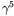had to have doubler fermions. On the face of it that seems to imply a stark choice between chiral symmetry and freedom from doublers.

There is, however, an interesting way around this apparent dilemma. This was discovered by Ginsparg and Wilson in a 1982 paper, were they studied the result of performing a spin-blocking step on a chirally symmetric continuum fermion action. What they discovered was that the Dirac operator of the blocked theory obeyed the anticommutation relation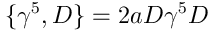now known as the Ginsparg-Wilson relation.

This relation has a number of interesting consequences: Firstly, it implies that the propagator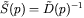obeys the anticommutation relation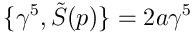and hence in coordinate space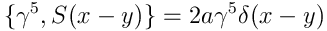i.e. the propagator is chirally invariant at all non-zero distances. Secondly, Lüscher discovered in 1998 that the Ginsparg-Wilson relation leads to a non-standard realization of chiral symmetry in the theory, which is invariant under the infinitesimal transformations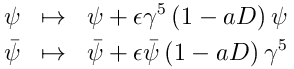The fermion measure, however, transforms anomalously under this symmetry, and a little calculation shows that this gives precisely the correct chiral anomaly.

On the other hand, since the Wilson operator no longer anticommutes with, the conditions of the Nielsen-Ninomiya theorem no longer apply, and there is hence no reason to expect the existence of any doubler fermions.

What all this means is that the correct chiral physics can be obtained from a lattice theory, provided one is able to find a solution to the Ginsparg-Wilson relation. The next post in this series will look at some of the fermion actions that arise from this.